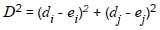# Dimensionality of Data

## Producer Field Guide

HGD_Product
Producer Field Guide
HGD_Portfolio_Suite
Producer

Spectral Dimensionality is determined by the number of sets of values being used in a process. In image processing, each band of data is a set of values. An image with four bands of data is said to be four-dimensional (Jensen, 1996).

The letter n is used consistently in this documentation to stand for the number of dimensions (bands) of image data.

Measurement Vector

The measurement vector of a pixel is the set of data file values for one pixel in all n bands. Although image data files are stored band-by-band, it is often necessary to extract the measurement vectors for individual pixels.

Measurement Vector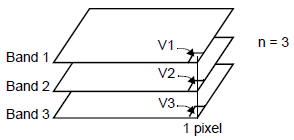According to the above figure:

i = particular band

Vi = data file value of the pixel in band i, then the measurement vector for this pixel is: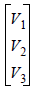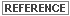See Matrix Algebra for an explanation of vectors.

Mean Vector

When the measurement vectors of several pixels are analyzed, a mean vector is often calculated. This is the vector of the means of the data file values in each band. It has n elements.

Mean Vector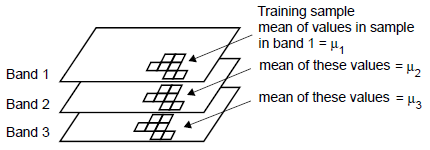According to Figure 255:

i = a particular band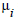= mean of the data file values of the pixels being studied, in band i, then the mean vector for this training sample is: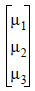Feature Space

Many algorithms in image processing compare the values of two or more bands of data. The programs that perform these functions abstractly plot the data file values of the bands being studied against each other. An example of such a plot in two dimensions (two bands) is illustrated in the following figure.

Two Band Plot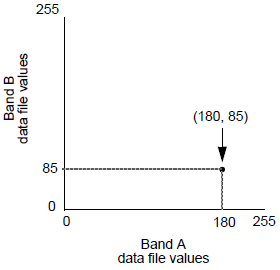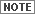If the image is 2-dimensional, the plot does not always have to be 2-dimensional.

In the above figure, the pixel that is plotted has a measurement vector of: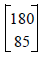The graph above implies physical dimensions for the sake of illustration. Actually, these dimensions are based on spectral characteristics represented by the digital image data. As opposed to physical space, the pixel above is plotted in feature space. Feature space is an abstract space that is defined by spectral units, such as an amount of electromagnetic radiation.

Feature Space Images

Several techniques for the processing of multiband data make use of a two-dimensional histogram, or feature space image. This is simply a graph of the data file values of one band of data against the values of another band.

Two-band Scatterplot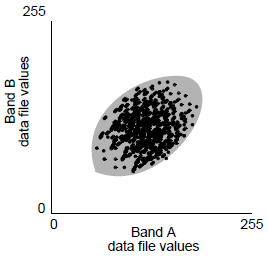The scatterplot pictured in the above figure can be described as a simplification of a two-dimensional histogram, where the data file values of one band have been plotted against the data file values of another band. This figure shows that when the values in the bands being plotted have jointly normal distributions, the feature space forms an ellipse.

This ellipse is used in several algorithms—specifically, for evaluating training samples for image classification. Also, two-dimensional feature space images containing ellipses are helpful to illustrate principal components analysis.See Enhancement for more information on principal components analysis, Classification for information on training sample evaluation, and Rectification for more information on orders of transformation.

n-Dimensional Histogram

If two-dimensional data can be plotted on a two-dimensional histogram, as above, then n-dimensional data can, abstractly, be plotted on an n-dimensional histogram, defining n-dimensional spectral space.

Each point on an n-dimensional scatterplot has n coordinates in that spectral space—a coordinate for each axis. The n coordinates are the elements of the measurement vector for the corresponding pixel.

In some image enhancement algorithms (most notably, principal components), the points in the scatterplot are replotted, or the spectral space is redefined in such a way that the coordinates are changed, thus transforming the measurement vector of the pixel.

When all data sets (bands) have jointly normal distributions, the scatterplot forms a hyperellipsoid. The prefix "hyper" refers to an abstract geometrical shape, that is defined in more than three dimensions.In this documentation, 2-dimensional examples are used to illustrate concepts that apply to any number of dimensions of data. The 2-dimensional examples are best suited for creating illustrations to be printed.

Spectral Distance

Euclidean Spectral distance is distance in n-dimensional spectral space. It is a number that allows two measurement vectors to be compared for similarity. The spectral distance between two pixels can be calculated as follows: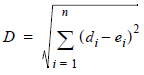Where:

D = spectral distance

n = number of bands (dimensions)

i = a particular band

di = data file value of pixel d in band i

ei = data file value of pixel e in band i

This is the equation for Euclidean distance—in two dimensions (when n = 2), it can be simplified to the Pythagorean Theorem (c2 = a2 + b2), or in this case: